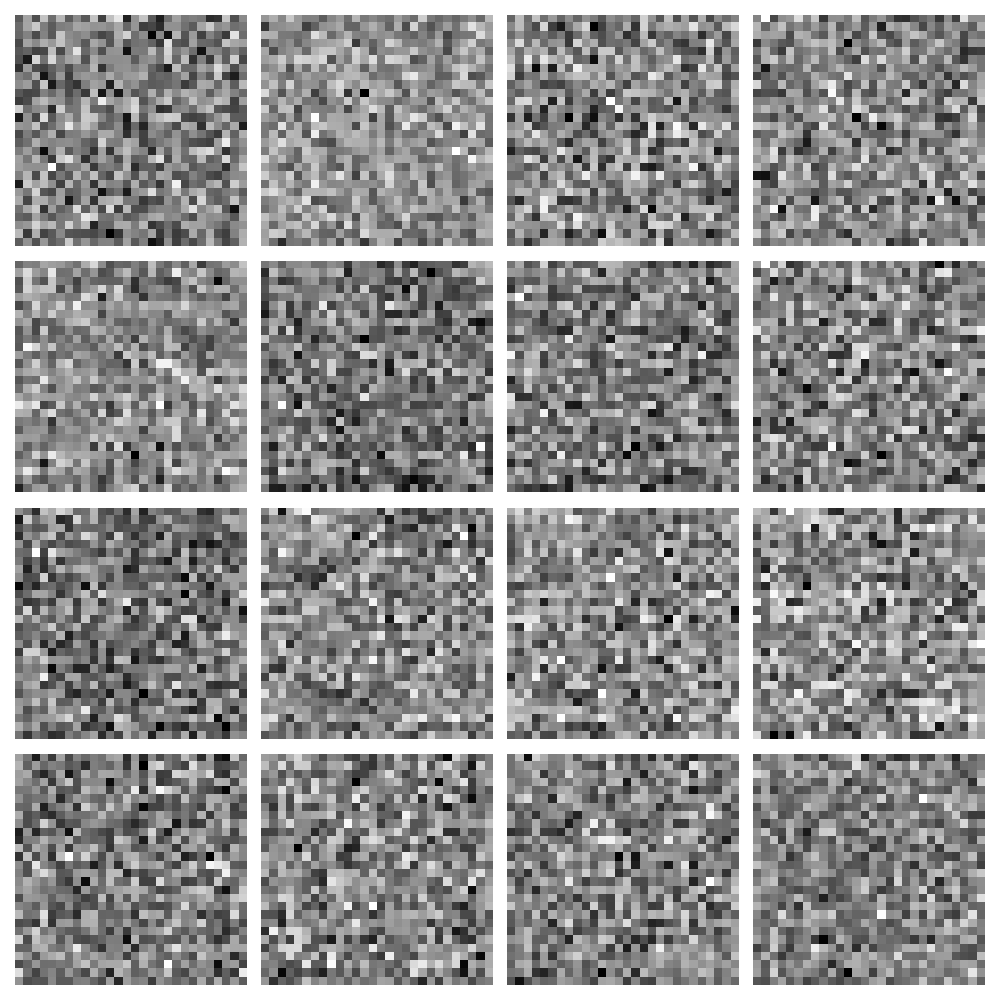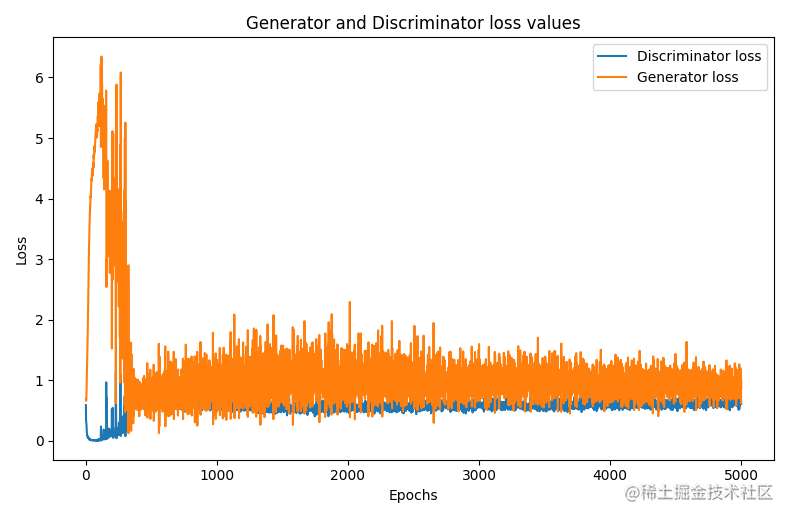# Keras深度學習——生成對抗網路

## 生成對抗網路詳解

`GAN` 包含兩個網路：生成器和鑑別器。生成器的目標是生成逼真的影象騙過鑑別器，鑑別器的目標是確定輸入影象是真實影象還是生成器生成的偽造影象。

## 利用生成對抗網路生成手寫數字影象

shape = (28, 28, 1) epochs = 5000 batch_size = 64 save_interval = 100 `接下來，定義生成器，對於生成器模型，其採用形狀為 `100` 維的噪聲向量，通過數個全連線層後生成 `28×28×1=1024` 的向量，最後將其整形為形狀為 `(28, 28, 1)` 的影象，在模型中使用 `LeakyReLU` 啟用函式。：`python def generator(): model = Sequential() model.add(Dense(256, input_shape=(100,))) model.add(LeakyReLU(alpha=0.2)) model.add(BatchNormalization(momentum=0.8)) model.add(Dense(512)) model.add(LeakyReLU(alpha=0.2)) model.add(BatchNormalization(momentum=0.8)) model.add(Dense(1024)) model.add(LeakyReLU(alpha=0.2)) model.add(BatchNormalization(momentum=0.8)) model.add(Dense(28281, activation='tanh')) model.add(Reshape(shape)) return model `生成器的簡要資訊輸出如下：`shell Model: "sequential"

# Layer (type) Output Shape Param #

dense (Dense) (None, 256) 25856

leaky_re_lu (LeakyReLU) (None, 256) 0

batch_normalization (BatchNo (None, 256) 1024

dense_1 (Dense) (None, 512) 131584

leaky_re_lu_1 (LeakyReLU) (None, 512) 0

batch_normalization_1 (Batch (None, 512) 2048

dense_2 (Dense) (None, 1024) 525312

leaky_re_lu_2 (LeakyReLU) (None, 1024) 0

batch_normalization_2 (Batch (None, 1024) 4096

dense_3 (Dense) (None, 784) 803600

# reshape (Reshape) (None, 28, 28, 1) 0

Total params: 1,493,520 Trainable params: 1,489,936 Non-trainable params: 3,584

`接下來，我們將構建鑑別器模型，該模型將形狀為 `(28, 28, 1)` 的輸入影象，併產生輸出 `1` 或 `0`，用於表示輸入影象是原始真實影象還是生成的偽造影象：`python def discriminator(): model = Sequential() model.add(Flatten(input_shape=shape)) model.add(Dense(1024)) model.add(LeakyReLU(alpha=0.2)) model.add(Dense(256)) model.add(LeakyReLU(alpha=0.2)) model.add(Dense(1, activation='sigmoid')) return model `鑑別器模型的簡要結構資訊輸出如下：`shell Model: "sequential_1"

# Layer (type) Output Shape Param #

flatten (Flatten) (None, 784) 0

dense_4 (Dense) (None, 1024) 803840

leaky_re_lu_3 (LeakyReLU) (None, 1024) 0

dense_5 (Dense) (None, 256) 262400

leaky_re_lu_4 (LeakyReLU) (None, 256) 0

# dense_6 (Dense) (None, 1) 257

Total params: 1,066,497 Trainable params: 1,066,497 Non-trainable params: 0

`編譯生成器和鑑別器模型：`python generator = generator() generator.compile(loss='binary_crossentropy', optimizer=Adam(lr=0.0002, beta_1=0.5, decay=8e-8))

discriminator = discriminator() discriminator.compile(loss='binary_crossentropy', optimizer=Adam(lr=0.0002, beta_1=0.5, decay=8e-8), metrics=['acc']) `組合生成器與鑑別器，定義 `GAN` 模型，該模型用於訓練生成器的權重，同時凍結鑑別器的權重。`GAN` 模型將隨機噪聲作為輸入，並使用生成器網路將該噪聲轉換為形狀為 `(28, 28, 1)` 的影象，然後模型預測生成的影象是真實影象還是偽造影象：`python def gan(discriminator, generator): discriminator.trainable = False model = Sequential() model.add(generator) model.add(discriminator) return model

gan = gan(discriminator, generator) gan.compile(loss='binary_crossentropy', optimizer=Adam(lr=0.0002, beta_1=0.5, decay=8e-8)) `定義函式用於繪製生成的影象：`python def plot_images(samples=16, step=0): noise = np.random.normal(0, 1, (samples, 100)) images = generator.predict(noise) plt.figure(figsize=(10, 10)) for i in range(images.shape): plt.subplot(4, 4, i + 1) image = images[i, :, :, :] image = np.reshape(image, [28, 28]) plt.imshow(image, cmap='gray') plt.axis('off') plt.tight_layout() plt.show() `載入 `MNIST` 資料集，並對資料集進行預處理：`python (x_train, ), (, _) = mnist.load_data()

x_train = (x_train.astype(np.float32) - 127.5) / 127.5 x_train = np.expand_dims(x_train, axis=3) ```因為 `GAN` 模型基於給定的影象集 `x_train` 生成新影象，因此我們不需要輸出標籤。 接下來，通過在多個 `epochs` 內訓練 `GAN` 來優化網路權重。 獲取真實影象 `legit_images` 並利用噪聲資料生成偽造影象 `synthetic_images`，使用噪聲資料 `gen_noise` 作為輸入，嘗試生成真實影象：```python disc_loss = [] gen_loss = []

for cnt in range(epochs): random_index = np.random.randint(0, len(x_train) - batch_size / 2) legit_images = x_train[random_index: random_index + batch_size // 2].reshape(batch_size // 2, 28, 28, 1) gen_noise = np.random.normal(-1, 1, (batch_size // 2, 100))/2 synthetic_images = generator.predict(gen_noise) `使用 `train_on_batch` 方法訓練鑑別器，`train_on_batch` 用於使用單個批資料對模型執行一次梯度更新，在輸出中，實際影象的值為 `1`，偽造影象的值為 `0`：`python x_combined_batch = np.concatenate((legit_images, synthetic_images)) y_combined_batch = np.concatenate((np.ones((batch_size // 2, 1)), np.zeros((batch_size // 2, 1)))) d_loss = discriminator.train_on_batch(x_combined_batch, y_combined_batch) `接下來，我們準備用於訓練生成器的資料，隨機噪聲作為輸入資料 `noise`，而 `y_mislabeled` 是用於訓練生成器的輸出，需要注意的是，這裡的輸出與訓練鑑別器時的輸出完全相反，即使用 `1` 作為偽造影象的值：`python noise = np.random.normal(-1, 1, (batch_size, 100))/2 y_mislabled = np.ones((batch_size, 1)) `接下來，我們訓練 `GAN` 模型，其中鑑別器權重被凍結，而生成器的權重會得到更新以最小化損失，生成器的任務是生成可欺騙鑑別器的影象，即令鑑別器輸出值 `1`：`python g_loss = stacked_generator_discriminator.train_on_batch(noise, y_mislabled) `然後，我們記錄各個 `epoch` 內的生成器損失和鑑別器損失，並按照指定間隔檢視生成器生成影象：`python g_loss = gan.train_on_batch(noise, y_mislabled) disc_loss.append(d_loss) gen_loss.append(g_loss) print('epoch: {}, [Discriminator: {}], [Generator: {}]'.format(cnt, d_loss, g_loss)) if cnt % 100 == 0: plot_images(step=cnt) ```